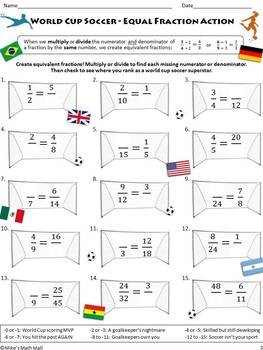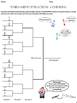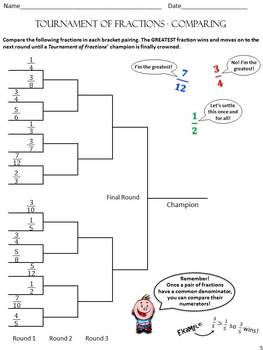digital

# Back to School Math Rust Remover for 5th GradeSubject
Resource Type
FormatsTpT Digital Activity

PDF (3 MB|13 pages)
Standards
\$4.75TpT Digital Activity Included
This resource has been prepared for device-based learning by the Teacher-Author and can be assigned to students via Google Classroom.

### Description

Back to School Math for 5th Grade - This is a super-fun, engaging, and practical collection of activities that will help incoming 5th graders recall important 4th grade math concepts and prepare them for the 5th grade math curriculum. The review pack will also help indicate individual student skill level.

Review Concepts:

Essential vocabulary

Adding, subtracting, multiplying, and dividing whole numbers

Equivalent fractions

Comparing fractions

Prime and composite numbers

Rounding whole numbers

Practice pages may be printed (in color or grey scale) or used with SmartBoards.

Answer Keys provided for all activities.

I Sincerely Hope this Helps! And Thank You!

Electronic distribution limited to single classroom use only.

Total Pages
13 pages
Included
Teaching Duration
1 Week
Report this Resource to TpT
Reported resources will be reviewed by our team. Report this resource to let us know if this resource violates TpT’s content guidelines.

### Standards

to see state-specific standards (only available in the US).
Find all factor pairs for a whole number in the range 1-100. Recognize that a whole number is a multiple of each of its factors. Determine whether a given whole number in the range 1-100 is a multiple of a given one-digit number. Determine whether a given whole number in the range 1-100 is prime or composite.
Find whole-number quotients of whole numbers with up to four-digit dividends and two-digit divisors, using strategies based on place value, the properties of operations, and/or the relationship between multiplication and division. Illustrate and explain the calculation by using equations, rectangular arrays, and/or area models.
Fluently multiply multi-digit whole numbers using the standard algorithm.
Find whole-number quotients and remainders with up to four-digit dividends and one-digit divisors, using strategies based on place value, the properties of operations, and/or the relationship between multiplication and division. Illustrate and explain the calculation by using equations, rectangular arrays, and/or area models.
Multiply a whole number of up to four digits by a one-digit whole number, and multiply two two-digit numbers, using strategies based on place value and the properties of operations. Illustrate and explain the calculation by using equations, rectangular arrays, and/or area models.

### Q & A

Teachers Pay Teachers is an online marketplace where teachers buy and sell original educational materials.